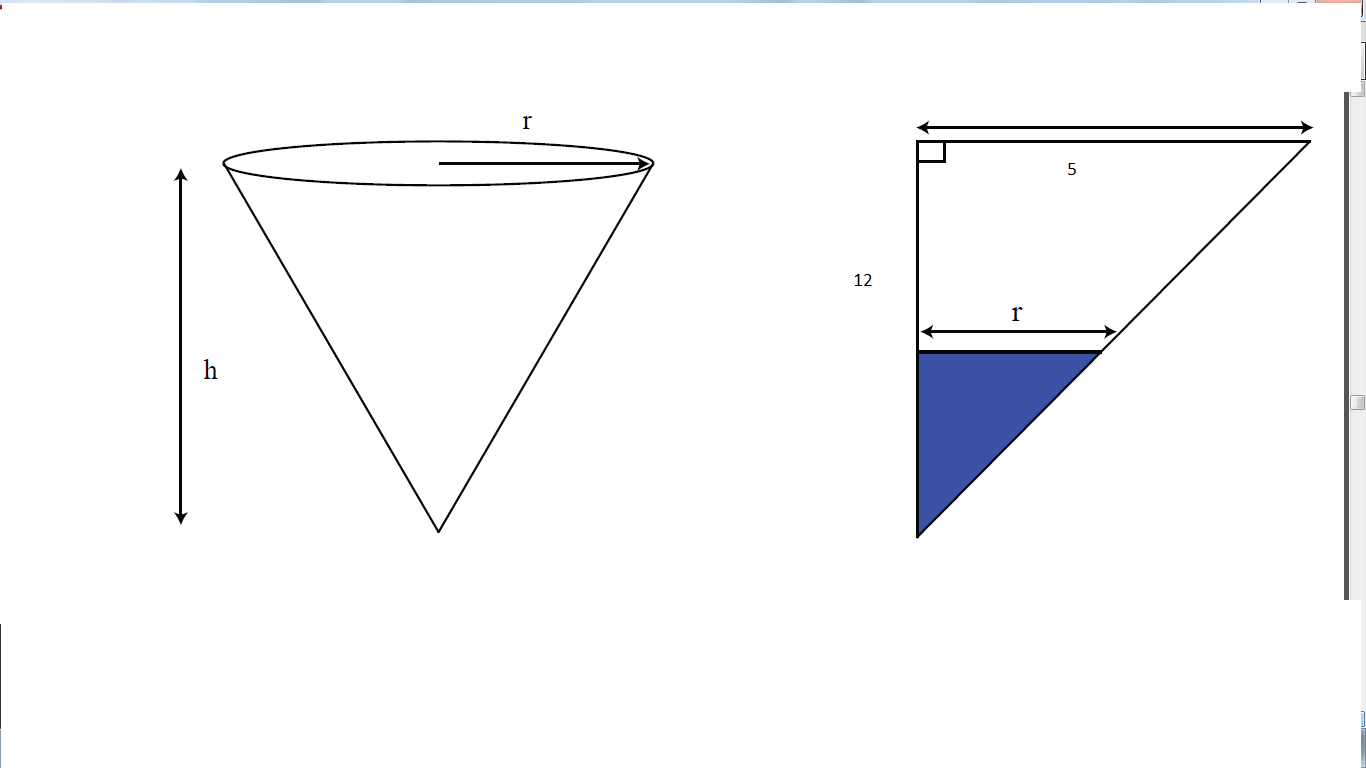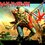# Related RatesThis note along with the problem set is based on basic applications of differentiation to real-world problems.To understand this note you need to have basic ideas of Differential Calculus.

This note is about problems on related rates-which is the study of change of one variable with respect to another provided that we find a constant parameter providing a relationship between those two variables.AN important point for approaching problems like these is to find that constant variable providing the link between the variables we want to evaluate.

$\textbf{Problem.}$Consider a conical tank. Its radius at the top is 5 feet, and it’s 12 feet high. It’s being filled with water at the rate of 3 cubic feet per minute. How fast is the water level rising when it is 7 feet high? (See the given figure)

$\textbf{Ans.}$

From the second figure by triangle similarity we have $\frac{r}{5} = \frac{h}{12}$ $r = 5 \frac{h}{12}$

Now we need to find the constant parameter.Clearly it is the rate of change of volume!!Since given $\dfrac{\text{d}V}{\text{d}t} = 2$. We have $V = \frac{1}{3}\pi r^{2} h$

We put the value of $r$ in terms of $h$ to get

$V = \frac{1}{3}\pi (5 \frac{h}{12})^{2} h$

$V = \frac{1}{3}\pi (5 \frac{h}{12})^{2} h$

$V = \frac{1}{3}\pi (25 \frac{h^3}{144})$

Now we differentiate both sides to get:

$\dfrac{\text{d}V}{\text{d}t} = \frac{1}{3}\pi (25*3 \frac{h^2}{144})\dfrac{\text{d}h}{\text{d}t}$

.Now we simply put the values of $h$ and $\dfrac{\text{d}V}{\text{d}t}$ to get the correct answer.

Now that you have learnt how to approach these situations try your hand at the problems.They are not that hard......After that you'll see derivatives everywhere.7 years, 4 months ago

This discussion board is a place to discuss our Daily Challenges and the math and science related to those challenges. Explanations are more than just a solution — they should explain the steps and thinking strategies that you used to obtain the solution. Comments should further the discussion of math and science.

When posting on Brilliant:

• Use the emojis to react to an explanation, whether you're congratulating a job well done , or just really confused .
• Ask specific questions about the challenge or the steps in somebody's explanation. Well-posed questions can add a lot to the discussion, but posting "I don't understand!" doesn't help anyone.
• Try to contribute something new to the discussion, whether it is an extension, generalization or other idea related to the challenge.

MarkdownAppears as
*italics* or _italics_ italics
**bold** or __bold__ bold
- bulleted- list
• bulleted
• list
1. numbered2. list
1. numbered
2. list
Note: you must add a full line of space before and after lists for them to show up correctly
paragraph 1paragraph 2

paragraph 1

paragraph 2

[example link](https://brilliant.org)example link
> This is a quote
This is a quote
    # I indented these lines
# 4 spaces, and now they show
# up as a code block.

print "hello world"
# I indented these lines
# 4 spaces, and now they show
# up as a code block.

print "hello world"
MathAppears as
Remember to wrap math in $$ ... $$ or $ ... $ to ensure proper formatting.
2 \times 3 $2 \times 3$
2^{34} $2^{34}$
a_{i-1} $a_{i-1}$
\frac{2}{3} $\frac{2}{3}$
\sqrt{2} $\sqrt{2}$
\sum_{i=1}^3 $\sum_{i=1}^3$
\sin \theta $\sin \theta$
\boxed{123} $\boxed{123}$

Sort by:

khanacademy.com also has very nice explanations of this.

- 7 years, 4 months ago

The problem says that the rate of filling is 3 cubic feet per minute, so why is $\frac{dv}{dt} = 2$?

- 7 years, 4 months ago

Oops...typo....Sorry for that....

- 7 years, 4 months ago

Nice explanation thanks

- 7 years, 4 months ago